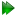Subsections

Components of the Ri'(θ) equations

To simplify the calculations of the gradients and Hessians the Ri'(θ) equations have been broken down into a number of components. These include the dipolar and CSA constants as well as the dipolar and CSA spectral density terms for each of the three transformed relaxation data types { R1, R2, σNOE}. The segregation of these components simplifies the maths as many partial derivatives of the components are zero.

Dipolar constant

The dipolar constant is defined as

 d =. (15.19)

This component of the relaxation equations is independent of the parameter of the spectral density function θj, the chemical exchange parameter ρex, and the CSA parameter Δσ. Therefore the partial and second partial derivatives with respect to these parameters is zero. Only the derivative with respect to the bond length r is non-zero being

 d'≡= -. (15.20)

The second derivative with respect to the bond length is

 d”≡=. (15.21)

CSA constant

The CSA constant is defined as

 c =. (15.22)

The partial derivative of this component with respect to all parameters but the CSA parameter Δσ is zero. This derivative is

 c'≡=. (15.23)

The CSA constant second derivative with respect to Δσ is

 c”≡=. (15.24)

Rex constant

The Rex constant is defined as

 Rex = ρex(2πωH)2. (15.25)

The partial derivative of this component with respect to all parameters but the chemical exchange parameter ρex is zero. This derivative is

 Rex'≡= (2πωH)2. (15.26)

The Rex constant second derivative with respect to ρex is

 Rex”≡= 0. (15.27)

Spectral density terms of the R1 dipolar component

For the dipolar component of the R1 equation (7.3a) on pagethe spectral density terms are

 JdR1 = J(ωH - ωX) + 3J(ωX) + 6J(ωH + ωX). (15.28)

The partial derivative of these terms with respect to the spectral density function parameter θj is

 JdR1′≡=+3+6. (15.29)

The second partial derivative with respect to the spectral density function parameters θj and θk is

 JdR1”≡=+3+6. (15.30)

Spectral density terms of the R1 CSA component

For the CSA component of the R1 equation (7.3a) on pagethe spectral density terms are

 JcR1 = J(ωX). (15.31)

The partial derivative of these terms with respect to the spectral density function parameter θj is

 JcR1′≡=. (15.32)

The second partial derivative with respect to the spectral density function parameters θj and θk is

 JcR1”≡=. (15.33)

Spectral density terms of the R2 dipolar component

For the dipolar component of the R2 equation (7.3b) on pagethe spectral density terms are

 JdR2 = 4J(0) + J(ωH - ωX) + 3J(ωX) + 6J(ωH) + 6J(ωH + ωX). (15.34)

The partial derivative of these terms with respect to the spectral density function parameter θj is

 JdR2′≡= 4++3+6+6. (15.35)

The second partial derivative with respect to the spectral density function parameters θj and θk isSpectral density terms of the R2 CSA component

For the CSA component of the R2 equation (7.3b) on pagethe spectral density terms are

 JcR2 = 4J(0) + 3J(ωX). (15.36)

The partial derivative of these terms with respect to the spectral density function parameter θj is

 JcR2′≡= 4+3. (15.37)

The second partial derivative with respect to the spectral density function parameters θj and θk is

 JcR2”≡= 4+3. (15.38)

Spectral density terms of the σNOE dipolar component

For the dipolar component of the σNOE equation (7.3c) on pagethe spectral density terms are

 JdσNOE = 6J(ωH + ωX) - J(ωH - ωX). (15.39)

The partial derivative of these terms with respect to the spectral density function parameter θj is

 JdσNOE′≡= 6-. (15.40)

The second partial derivative with respect to the spectral density function parameters θj and θk is

 JdσNOE”≡= 6-. (15.41)

The relax user manual (PDF), created 2020-08-26.